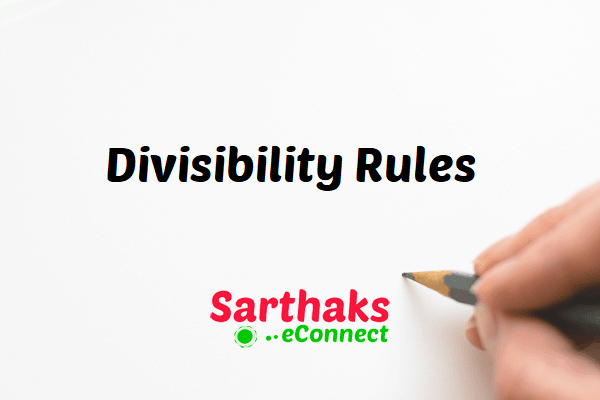## Divisibility Rules:

### Divisibility by 2 :

A number is divisible by 2 if its unit’s digit is even or 0.

### Divisibility Rule for 3 :

A number is divisible by 3 if the sum of its digits are divisible by 3.

### Divisibility Rule for 4 :

A number is divisible by 4 if the last 2 digits are divisible by 4, or if the last two digits are 0’s.

### Divisibility Rule for 5 :

A number is divisible by 5 if its unit’s digit is 5 or 0.

### Divisibility Rule for 6 :

A number is divisible by 6 if it is simultaneously divisible by 2 and 3.

### Divisibility Rules for 7 :

A number is divisible by 7 if unit’s place digit is multiplied by 2 and subtracted from the remaining digits and the number obtained is divisible by 7.
For example,

1680 7 = 1680 – 7 × 2 = 1666
It is difficult to decide whether 1666 is divisible by 7 or not. In such cases, we continue the process again and again till it becomes easy to decide whether the number is divisible by 7 or not.
166 6 → 166 – 6 x 2 = 154
Again 15 4 → 15 – 4 x 2 = 7, divisible by 7
Hence 16807 is divisible by 7.

### Divisibility Rule for 8 :

A number is divisible by 8 if the last 3 digits of the number are divisible by 8, or if the last three digits of a number are zeros.

### Divisibility Rule for 9 :

A number is divisible by 9 if the sum of its digits is divisible by 9.

### Divisibility by 10 :

A number is divisible by 10 if its unit’s digit is 0.

### Divisibility Rules for 11 :

A number is divisible by 11 if the sum of digits at odd and even places are equal or differ by a number divisible by 11.

### Divisibility by 12 :

A number is divisible by 12 if the number is divisible by both 4 and 3.

### Divisibility Rules for 13 :

A number is divisible by 13 if its unit’s place digit is multiplied by 4 and added to the remaining digits and the number obtained is divisible by 13.
For example,
219 7 → 219 + 7 x 4 = 247
Again 24 7 → 24 + 7 x 4 = 52, divisible by 13.
Hence 2197 is divisible by 13.

### Divisibility by 14 :

A number is divisible by 14 if the number is divisible by both 2 and 7.

### Divisibility by 15 :

A number is divisible by 15 if the number is divisible by both 3 and 5.

### Divisibility by 16 :

A number is divisible by 16 if its last 4 digits is divisible by 16 or if the last four digits are zeros.

### Divisibility Rules for 17 :

A number is divisible by 17 if its unit’s place digit is multiplied by 5 and subtracted from the remaining digits and the number obtained is divisible by 17.
For example,
491 3 → 491- 3 x 5 = 476
Again, 47 6 → 47 – 6 x 8 =17, divisible by 17.
Hence 4913 is divisible by 17.

### Divisibility by 18 :

A number is divisible by 18 if the number is divisible by both 2 and 9.

### Divisibility Rules for 19 :

A number is divisible by 19 if its unit’s place digit is multiplied by 2 and added to the remaining digits and the number obtained is divisible by 19.
For example,
4873 7 → 4873+ 7 x 2 = 4887
488 7 → 488 + 7 x 2 = 502
50 2 → 50 + 2 x 2 = 54 not divisible by 19.
Hence 48737 is not divisible by 19.

## Properties of Divisibility:

(i) The product of 3 consecutive natural numbers is divisible by 6.
(ii) The product of 3 consecutive natural numbers, the first of which is even, is divisible by 24.
(iii) Difference between any number and the number obtained by writing the digits in reverse order is divisible by 9.
(iv) Any number written in the form (10^n – 1) is divisible by 3 and 9.
(v) Any number in the form abcabc (a, b, c are three different digits) is divisible by 1001.
(vi) (a) (a^n – b^n) is divisible both by (a + b) and (a – b), when n is even.
(b) (a^n – b^n) is divisible only by (a – b), when n is odd.